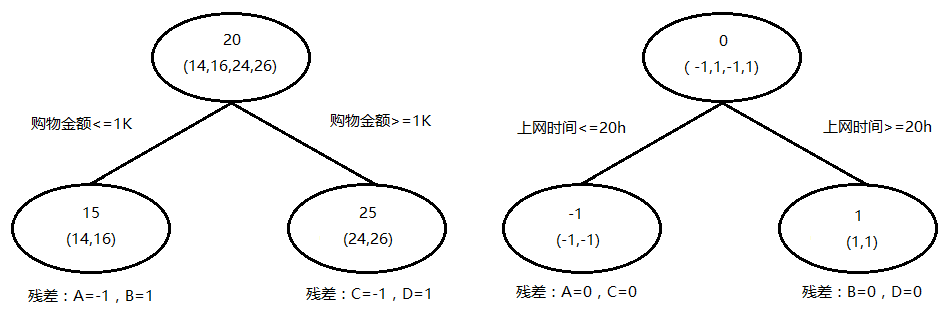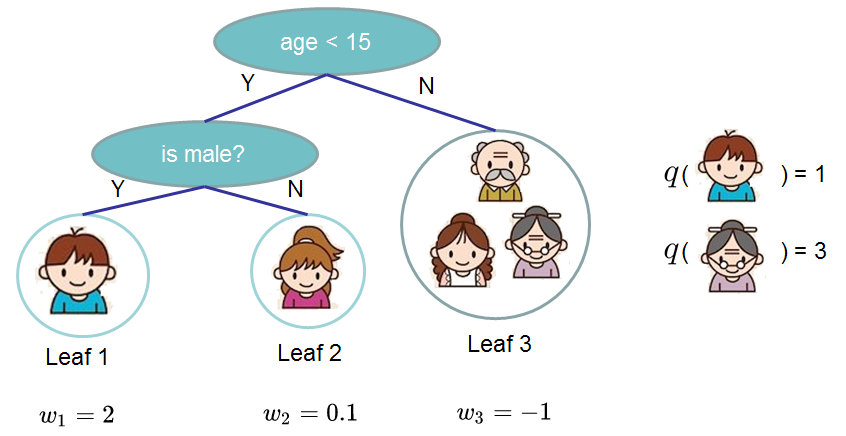## 前言

• 对集成学习进行了简要的说明
• 针对GBDT的学习过程进行了简要介绍
• 针对Xgboost的损失函数进行了简要介绍

## 集成学习

• 分类
根据个体学习器的生成方式，目前的集成学习方法大致可以分为两大类，即个体学习器间存在强依赖关系、必须串行生成的序列化方法，以及个体学习器间不存在强依赖关系、可同时生成的并行化方法；前者的代表是Boosting，后者的代表是Bagging和随机森林。

• 结合策略
对于基分类器最终的结合策略常见的方法有如下几种：

• 平均法
对于数值形输出，最常见的结合策略即为平均法：

$H(x)=\frac{1}{T}\sum_{i=1}^{T}h_{i}(x)$

其中$h_{i}(x)$为基学习器的输出结果，$H(x)$为最终学习器的结果，$T$为基学习器的个数。

• 加权平均法

$H(x)=\sum_{i=1}^{T}w_{i}h_{i}(x)$

其中$w_{i}$是个体学习器$h_{i}$的权重，通常要求$w_{i}\geqslant 0,\sum_{i=1}^{T}w_{i}=1$。显然，简单平均法是加权平均法令$w_{i}=1/T$的特例。

• 投票法
预测结果为得票最多的标记，若同时有多个标记获得相同的票数，则从中随机选取一个。

• 学习法
当训练数据很多时，可以通过另一个学习器来对所有基学习器产生结果的结合方法进行学习，这时候个体学习器称为初级学习器，用于结合的学习器成为次级学习器或元学习器。

### 举例

$D = \{(\vec{x_{i}}, y_{i}),\ i\in[1, m]\}$ 训练集，共m个样本
$T$ 训练轮数
$D_{t}(x)$ $t$轮时样本的权重分布
$h_{t}$ $t$轮得到的基分类器
$\alpha_{t}$ $t$轮得到的基分类器的权重
$\epsilon_{t}$ $t$$h_{t}$的错误率
$P_{A}(D)$ 强分类器$A$在数据集$D$上的最终准确率

$x$ 0 1 2 3 4 5 6 7 8 9
$y$ 1 1 1 -1 -1 -1 1 1 1 -1

#### 第一轮

• $D_{1}(x)$
因为是第一轮，故所有样本的权重相同：

$D_{1}(\vec{x})=\{\frac{1}{10},\frac{1}{10},\frac{1}{10},\frac{1}{10},\frac{1}{10},\frac{1}{10},\frac{1}{10},\frac{1}{10},\frac{1}{10},\frac{1}{10}\}$

• $\theta$
因为是离散数据，所以$\theta$可以依次取0.5，1.5，2.5，...，8.5来对x进行分类，这里可以有两种分类方法：

• $x<\theta$时分为正类，$x>\theta$时分为负类，分类错误率对应$\epsilon_{t}^{1}$
• $x>\theta$时分为正类，$x<\theta$时分为负类，分类错误率对应$\epsilon_{t}^{2}$

最终要选择一个令$\epsilon_{1}$取得最小值的$\theta$与分类方法，这9个值在两种分类方法下，此层$h_{1}$的错误率$\vec{\epsilon_{1}}$分别为：

$\vec{\epsilon_{1}^{1}}=\{0.5*\frac{1}{10}, 0.4*\frac{1}{10}, 0.3*\frac{1}{10}, 0.4*\frac{1}{10}, 0.5*\frac{1}{10}, \\ 0.6*\frac{1}{10}, 0.5*\frac{1}{10}, 0.4*\frac{1}{10}, 0.3*\frac{1}{10}\}\\ \vec{\epsilon_{1}^{2}}=\{0.5*\frac{1}{10}, 0.6*\frac{1}{10}, 0.7*\frac{1}{10}, 0.6*\frac{1}{10}, 0.5*\frac{1}{10}, \\ 0.4*\frac{1}{10}, 0.5*\frac{1}{10}, 0.6*\frac{1}{10}, 0.7*\frac{1}{10}\}$

• $\alpha_{1}$
第一层基分类器$h_{1}$的权重$\alpha_{1}$的计算推到方法后面的推导部分再细说，此处只要知道通过如下的公式来计算即可：

$\alpha_{1}=\frac{1}{2}log\frac{1-\epsilon_{1}}{\epsilon_{1}}=0.4236$

• $H(x), P_{A}(D)$
根据如下公式计算$H(x)$，此时$T$为1：

$H(x) = \sum_{t=1}^{T}\alpha_{t}h_{t}(x)\\ H(x) =\{0.424, 0.424, 0.424, -0.424, -0.424, -0.424, -0.424, -0.424, -0.424, -0.424\}\\ sign(H(x)) = \{1, 1, 1, -1, -1, -1, -1, -1, -1, -1\}$

$P_{A}(D)=0.7$

#### 第二轮

• $D_{2}(x)$
第一轮训练完成后对$D_{1}(x)$进行更新得到$D_{2}(x)$，更新公式的推导过程也是等到后边的推到部分再说，此处还是只要知道通过下边的公式来计算更新即可：

$D_{2}(x)=\frac{D_{1}(x){e}^{-\alpha_{1}y(x)h_{1}(x)}}{Z_{1}}$

$D_{2}(x)=\{0.071, 0.071, 0.071, 0.071, 0.071, 0.071, 0.167, 0.167, 0.167, 0.071\}$

• $\theta$
我们依然对$\theta$依次取0.5, 1.5, ... , 8.5来对x进行分类，注意我们刚才已经得到了$D_{2}(x)$，样本权重的分布不再是第一轮全部相等的$\frac{1}{10}$了，如当$\theta$取0.5按第一种分类方法进行分类时，$\epsilon_{2}^{1}$计算方法如下：

$\epsilon_{2}^{1}(\theta=0.5) = 0*0.071+1*0.071+1*0.071+0*0.071+0*0.071+\\ 0*0.071+1*0.167+1*0.167+1*0.167+0*0.071\\ =0.643$

$\vec{\epsilon_{2}^{1}}=\{0.643, 0.571, 0.5, 0.571, 0.643, 0.714, 0.548, 0.381, 0.214\}\\ \vec{\epsilon_{2}^{2}}=\{0.357, 0.429, 0.5, 0.429, 0.357, 0.286, 0.452, 0.619, 0.786\}$

• $\alpha_{2}$
依然使用如下公式进行计算：

$\alpha_{1}=\frac{1}{2}log\frac{1-\epsilon_{2}}{\epsilon_{2}}=0.6496$

• $H(x), P_{A}(D)$
继续根据如下公式计算$H(x)$，此时$T$为2：

$H(x) = \sum_{t=1}^{T}\alpha_{t}h_{t}(x)\\ H(x) =\{1.073, 1.073, 1.073, 0.226, 0.226, 0.226, 0.226, 0.226, 0.226, -1.073\}\\ sign(H(x)) = \{1, 1, 1, 1, 1, 1, 1, 1, 1, -1\}$

$P_{A}(D)=0.7$

#### 第三轮

• $D_{3}(x)$
使用$D_{2}(x)$更新得到$D_{3}(x)$如下：

$D_{3}(x)=\{0.045, 0.045, 0.045, 0.167, 0.167, 0.167, 0.106, 0.106, 0.106, 0.045\}$

• $\theta$
继续使用之前的方法得到$\vec{\epsilon_{3}^{1}}$$\vec{\epsilon_{3}^{2}}$分别为：

$\vec{\epsilon_{3}^{1}}=\{0.409, 0.364, 0.318, 0.485, 0.652, 0.818, 0.712, 0.606, 0.5\}\\ \vec{\epsilon_{3}^{2}}=\{0.591, 0.636, 0.682, 0.515, 0.348, 0.182, 0.288, 0.394, 0.5\}$

• $\alpha_{2}$
依然使用如下公式进行计算：

$\alpha_{1}=\frac{1}{2}log\frac{1-\epsilon_{2}}{\epsilon_{2}}=0.752$

• $H(x), P_{A}(D)$
继续根据如下公式计算$H(x)$，此时$T$为3：

$H(x) = \sum_{t=1}^{T}\alpha_{t}h_{t}(x)\\ H(x) =\{0.321, 0.321, 0.321, -0.526, -0.526, -0.526, 0.978, 0.978, 0.978, -0.321\}\\ sign(H(x)) = \{1, 1, 1, -1, -1, -1, 1, 1, 1, -1\}$

$P_{A}(D)=1$

• 在第$t$轮被分错的样本，在下一轮更新得到的$D_{t+1}(x)$中权值将被增大
• 在第$t$轮被分对的样本，在下一轮更新得到的$D_{t+1}(x)$中权值将被减小
• 所使用的决策树桩总是选取让错误率$\epsilon_{t}$（所有被$h_{t}$分类错误的样本在$D_{t}(x)$中对应的权值之和）最低的阈值来设计基本分类器
• 最终Adboost得到的$H(x)$为：

$sign(H(x))=sign(0.4236*h_{1}+0.6496*h_{2}+0.752*h_{3})$

#### 其他情况

• 分类问题
• 决策树桩
见上例
• 决策树
每一个节点的选择过程都需要将$D_{t}(x)$考虑进去，即在定义损失函数的时候，考虑每一个样本被分错的代价（权重）
• 逻辑回归
阈值$\theta$的选取需令本层$\epsilon_{t}$取得最小值
• 回归问题
• 按照$D_{t}(x)$的分布对原始数据集进行重新采样，利用采样后得到的新数据集再进行训练

#### 推导

AdaBoost算法可以认为是一种模型为加法模型、损失函数为指数函数、学习算法为前向分步算法的而分类学习方法。在对$\alpha_{t}$$D_{t}$进行推导前，我们先对加法模型和前向分步算法进行简要介绍。

• 加法模型
加法模型的定义如下：

$f(x)=\sum_{M}^{m=1}\beta_{m}b(x;\gamma_{m})$

$\underset{\beta_{m},\gamma_{m}}{min}\sum_{i=1}^{N}L(y_{i},\sum_{M}^{m=1}\beta_{m}b(x;\gamma_{m}))$

• 前向分步算法
前向分步算法对加法模型的求解思路是：如果能够从前向后，每一步只学习一个基函数及其系数，逐步逼近优化目标，那么就可以简化优化的复杂度。即每步只需优化如下损失函数：

$\underset{\beta,\gamma}{min}\sum_{M}^{m=1}L(y_{i},\beta b(x;\gamma))$

• $\alpha_{t}$的推导过程

$H(x) = \sum_{t=1}^{T}\alpha_{t}h_{t}(x)$

$L(y, H(x)) = e^{-yH(x)}$

$H_{t}(x)=H_{t-1}(x)+\alpha_{t}h_{t}(x)$

$(\alpha_{t},h_{t}(x))=\underset{\alpha_{t},h_{t}}{arg\ min}\sum_{i=1}^{N}e^{-y_{i}(H_{t-1}(x)+\alpha_{t}h_{t}(x))}\\ =\underset{\alpha_{t},h_{t}}{arg\ min}\sum_{i=1}^{N}\bar{w}_{ti}e^{-y_{i}\alpha_{t}h_{t}(x)}\\ h_{t}(x)^{*}=\underset{h_{t}(x)}{arg\ min}\sum_{i=1}^{N}\bar{w}_{ti}I(y_{i}\neq h_{t}(x))$

$\sum_{i=1}^{N}\bar{w}_{ti}e^{y_{i}\alpha_{t}h_{t}(x_{i})}\\ =\sum_{y_{i}=h_{t}(x_{i})}\bar{w}_{ti}e^{-\alpha_{t}}+\sum_{y_{i}\neq h_{t}(x_{i})}\bar{w}_{ti}e^{\alpha_{t}}\\ =(e^{\alpha_{t}}-e^{-\alpha_{t}})\sum_{i=1}^{N}\bar{w}_{ti}I(y_{i}\neq h_{t}(x_{i}))+e^{-\alpha}\sum_{i=1}^{N}\bar{w}_{ti}$

$\alpha_{t}^{*}=\frac{1}{2}log\frac{1-e_{t}}{e_{t}}$

$e_{t}=\frac{\sum_{i=1}^{N}\bar{w}_{ti}I(y_{i}\neq h_{t}(x_{i}))}{\sum_{i=1}^{N}\bar{w}_{ti}}\\ =\sum_{i=1}^{N}w_{ti}I(y_{i}\neq h_{t}(x_{i}))$

• $D_{t}$的推导过程
接下来我们对$D_{t}$的更新公式进行推导。由之前推导过程中所得到的下边两个式子：

$H_{t}(x)=H_{t-1}(x)+\alpha_{t}h_{t}(x)\\ \bar{w}_{ti}=e^{-y_{i}h_{t}(x_{i})}$

$\bar{w}_{t+1,i}=\bar{w}_{t,i}e^{-y_{i}\alpha_{t}h_{t}(x)}$

### GBDT

• 简介
GBDT即梯度提升树，提升方法依然采用的是加法模型与前向分布算法。以决策树为基函数的提升方法称为提升树。对分类问题决策树是二叉分类树，对回归问题决策树是二叉决策树。例如前文中的例子中所使用的决策树桩即为一个根节点直接连接两个叶节点的简单决策树。

• 学习过程
针对不同问题的提升树学习算法，其主要区别在于使用的损失函数不同。包括用平方误差损失函数的回归问题，是指数损失函数的分类问题，以及用一般损失函数的一般决策问题。对于分类问题，GBDT实质是把它转化为回归问题。在多分类问题中，假设有k个类别，那么每一轮迭代实质是构建了k棵树，对某个样本x的预测值为

$f_{1}(x),f_{2}(x),...,f_{k}(x)$

依然采用前向分步算法，过程如下：
$$f_{0}(x)=0\\ f_{m}(x)=f_{m-1}(x)+T(x;\Theta), m=1,2,...,M\\ f_{M}(x)=\sum_{m=1}^{M}T(x;\Theta_{m})$$

$$\hat{\Theta}_{m}=\underset{\Theta_{m}}{arg\ min}\sum_{i=1}^{N}L(y_{i},f_{m-1}(x_{i})+T(x_{i};\Theta_{m}))$$

$$L(y,f(x))=(y-f(x))^{2}$$

$$L(y,f_{m-1}(x)+T(x;\Theta_{m}))\\ =[y-f_{m-1}(x)-T(x;\Theta_{m})]^{2}\\ =[r-T(x;\Theta_{m})]^{2}$$

$$r=y-f_{m-1}(x)$$


• 举例
我们以简单的年龄预测为例，训练集共包括4个人：A,B,C,D，他们的年龄分别是14，16，24，26。每个人都有两个属性：每周的购物金额、每周的上网时间。数据集如下：

• A：购物金额<=1K，上网时间<=20h
• B：购物金额<=1K，上网时间>=20h
• C：购物金额>=1K，上网时间<=20h
• D：购物金额>=1K，上网时间>=20h

我们的目的就是构建一个GBDT模型来预测年龄。简单起见，我们限定每棵树只有一个分支，如下图所示：• 第一轮（树）
第一颗树节点属性的选取我们用最小化残差的方法。当选择每周购物金额作为划分属性时，两边叶子节点的残差和为：

$(14-\frac{14+16}{2})^2+(16-\frac{14+16}{2})^2+\\ (24-\frac{24+26}{2})^2+(26-\frac{24+26}{2})^2=4$

$(14-\frac{14+24}{2})^{2}+(24-\frac{14+24}{2})^{2}+\\ (16-\frac{16+26}{2})^{2}+(16-\frac{16+26}{2})^{2}=100$

• 第二轮（树）
第二颗树的目的是尽量去拟合由第一颗树产生的残差（-1，1，-1，1），特征选取的过程同第一棵树，不再复述。现在我们用每周的上网时间来作为划分的属性，则结果如上图第二颗树。A、C被划分到左节点，B、D被划分到右节点，两个节点的均值分别为-1和1。此时两个子节点的残差都为0，训练结束。

• 结果
不考虑泛化性，我们简单地使用训练集进行测试。
A = 15 + (–1) = 14
B = 15 + 1 = 16
C = 25 + (–1) = 24
D = 25 + 1 = 26
全部预测正确，厉(fei)害(hua)了我的哥。

• 总结

• GBDT每一轮训练时所关注的重点是本轮产生结果的残差，下一轮以本轮残差作为输入，尽量去拟合这个残差，使下一轮输出的残差不断变小。所以GBDT可以做到每一轮一定向损失函数减小的梯度方向变化，而传统的boosting算法只能是尽量向梯度方向减小，这是GBDT与传统boosting算法最大的区别，这也是为什么GBDT相比传统boosting算法可以用更少的树个数与深度达到更好的效果。
• 抽象地说，模型的训练过程是对一任意可导目标函数的优化过程，通过反复地选择一个指向负梯度方向的函数，该算法可被看作在函数空间里对目标函数进行优化。
• 回归问题
1. 用回归树去拟合残差，其实就是用回归树去拟合目标方程关于f（x）的梯度。
2. 回归的目标函数并不一定会用square loss。square loss的优点是便于理解和实现，缺点在于对于异常值它的鲁棒性较差，一个异常值造成的损失由于二次幂而被过分放大，会影响到最后得到模型在测试集上的表现。可以算则Absolute loss或者Huber loss代替。
• 分类问题
1. 此时的目标函数常用log loss，如KL-散度或者交叉熵。
2. 除了损失函数的区别外，分类问题和回归问题的区别还在于当多分类问题时，每轮可能会训练多个分类器。
• 由于决策树是非线性的，并且随着深度的加深，非线性越来越强，所以基于决策树的GBDT也是非线性的。

### xgboost

• 简介

GBDT算法只利用了一阶的导数信息，xgboost对损失函数做了二阶的泰勒展开，并在目标函数之外加入了正则项对整体求最优解，用以权衡目标函数的下降和模型的复杂程度，避免过拟合。所以不考虑细节方面，两者最大的不同就是目标函数的定义，接下来就着重从xgboost的目标函数定义上来进行介绍。

• 目标函数
xgboost的目标函数定义如下：

$Obj^{(t)}=\sum_{i=1}^{n}l(y_{i},\hat{y}_{i}^{(t)})+\sum_{i=1}^{t}\Omega(f_{i})\\ =\sum_{i=1}^{n}l(y_{i},\hat{y}_{i}^{(t-1)}+f_{t}(x_{i}))+\Omega(f_{t})+constant$

$f(x+\bigtriangleup x)\simeq f(x)+f^{'}(x)\bigtriangleup x+ \frac{1}{2}f^{''}(x)\bigtriangleup x^{2}$

$Obj^{(t)}\simeq \sum_{i=1}^{n}[l(y_{i},\hat y_{i}^{(t-1)})+g_{i}f_{t}(x_{i})+\frac{1}{2}h_{i}f_{t}^{2}(x_{i})]+\Omega(f_{t})+constant\\ g_{i}=\partial _{\hat y(t-1)}l(y_{i},\hat y^{t-1}),h_{i}=\partial _{\hat y(t-1)}^{2}l(y_{i},\hat y^{t-1})$

$Obj^{(t)}\simeq \sum_{i=1}^{n}[g_{i}f_{t}(x_{i})+\frac{1}{2}h_{i}f_{t}^{2}(x_{i})]+\Omega(f_{t})$

$f_{t}(x)=w_{q}(x)$

$\Omega(f_{t})=\gamma T+\frac{1}{2}\lambda \sum_{j=1}^{T}w_{j}^{2}$$Obj^{(t)}\simeq \sum_{i=1}^{n}[g_{i}f_{t}(x_{i})+\frac{1}{2}h_{i}f_{t}^{2}(x_{i})]+\Omega(f_{t})\\ =\sum_{i=1}^{n}[g_{i}w_{q}(x_{i})+\frac{1}{2}h_{i}w_{q}^{2}(x_{i})]+\gamma T+\frac{1}{2}\lambda \sum_{j=1}^{T}w_{j}^{2}\\ =\sum_{j=1}^{T}[(\sum_{i\subset I_{j}}g_{i})w_{j}+\frac{1}{2}(\sum_{i\subset I_{j}}h_{i}+\lambda)w_{j}^{2}]+\gamma T$

$G_{i}=\sum_{i\subset I_{j}}g_{i},\ H_{j}=\sum_{i\subset I_{j}}h_{i}$

$Obj^{(t)}=[G_{j}w_{j}+\frac{1}{2}(H_{j}+\lambda)w_{j}^{2}]+\gamma T$

$w_{j}^{*}=-\frac{G_{j}}{H_{j}+\lambda}$

$Gain=\frac{1}{2}[\frac{G_{L}^{2}}{H_{L}+\lambda}+\frac{G_{R}^{2}}{H_{R}+\lambda}-\frac{G_{L}^{2}+G_{R}}{H_{L}+H_{R}+\lambda}]-\gamma$

• 这个公式形式上跟ID3算法（采用entropy计算增益） 、CART算法（采用gini指数计算增益） 是一致的，都是用分裂后的某种值减去分裂前的某种值，从而得到增益

• 引入分割不一定会使情况变好，因为最后有一个新叶子的惩罚项。所以这也体现了预减枝的思想，即当引入的分割所带来的增益小于一个阈值时，就剪掉这个分割

• 上式中还有一个系数$\lambda$，是正则项里$w$的L2模平方的系数，对$w$做了平滑，也起到了防止过拟合的作用，这个是传统GBDT里不具备的特性

• 总结

### 代码

import math

def __init__(self, x_list = [0, 1, 2, 3, 4, 5, 6, 7, 8, 9],
y_list = [1, 1, 1, -1, -1, -1, 1, 1, 1, -1]):
"""initialization

input
x_list : training data set
y_list : labels of the x_list
"""
self.x_list = x_list
self.y_list = y_list
self.sample_count = len(self.y_list)
self.dt_list = [0.1] * self.sample_count  #the distribution of sample weights in the current round
self.dt_next_list = []  #the distribution of sample weights in the next round
self.ht_list = []  #results of the base classifier in the current round
self.alpha = 0.0  #the weight of the self.ht_list
self.Ht_list = [0.0] * self.sample_count  #results of the Adboost  in the current round
self.Ht_sign_list = []  #sign(self.Ht_list)
self.acc = 0.0  #the accuracy rate of the Adboost in the current round

def __get_decision_stump(self):
"""base classifier(decision stump)
obtain the decision stump by minimizing the classification error of current round with the self.dt_list

output
error_list  :  the error of each theta value and method
method_flag :  method flag
error_min   :  the minimum error of the error_list
theta_min   :  the value of theta of the corresponding error_min

error_min is necessary for return to compute other parameters, the rest are not
"""

def __get_ht_list(theta):
ht_list = []
for x in self.x_list:
if x < theta:
ht_list.append(1)
else:
ht_list.append(-1)
return ht_list

theta_list = [x + 0.5 for x in self.x_list[:-1]]
error_list = [[], []]
error_min = 99999
for theta in theta_list:
ht_list_1 = __get_ht_list(theta) #method 1
ht_list_2 = [-ht for ht in ht_list_1] #method 2
for method, ht_list in enumerate([ht_list_1, ht_list_2]):
error = 0
for loc, y_ in enumerate(ht_list):
if y_ != self.y_list[loc]:
error += self.dt_list[loc]
error_list[method].append(error)
if error < error_min:
error_min = error
theta_min = theta
method_flag = method + 1
self.ht_list = ht_list
return error_list, method_flag, error_min, theta_min

def __get_alpha(self, error):
self.alpha = 0.5 * math.log((1-error)/(error))

def __get_dt_next(self):
for loc, dt in enumerate(self.dt_list):
z = math.e**(-self.alpha * self.y_list[loc] * self.ht_list[loc])
self.dt_next_list.append(dt * z)
self.dt_next_list = [dt / sum(self.dt_next_list) for dt in self.dt_next_list]

def __get_acc_rate(self):
error = 0
for loc, _ in enumerate(self.x_list):
self.Ht_list[loc] += self.alpha * self.ht_list[loc]
self.Ht_sign_list = [int(math.pow(-1, (Ht > 0) * 1 + 1)) for Ht in self.Ht_list]
self.acc = map(cmp, self.Ht_sign_list, self.y_list).count(0) / (self.sample_count + 0.0)

def train(self, T = 3):
for t in range(T):
error_list, method, error, theta = self.__get_decision_stump()
self.__get_alpha(error)
self.__get_dt_next()
self.__get_acc_rate()

print '--------------Round %s--------------' % (t+1)
print 'error list : %s\n\t\t\t %s' % ([round(e, 3) for e in error_list],\
[round(e, 3) for e in error_list])
print 'theta : %s' % theta
print 'method : %s' % method
print 'error : %s' % round(error, 4)
print 'alpha : %s' % round(self.alpha, 4)
print 'ht_list : %s' % self.ht_list
print 'dt_list : %s' % [round(dt, 3) for dt in self.dt_list]
print 'dt_next_list : %s' % [round(dt, 3) for dt in self.dt_next_list]
print 'Ht_list : %s' % [round(Ht, 3) for Ht in self.Ht_list]
print 'Ht_sign_list : %s' % self.Ht_sign_list
print 'accuracy rate : %s' % self.acc
print '------------------------------------\n'
self.dt_list = self.dt_next_list
self.dt_next_list = []

if self.acc == 1.0:
break

if __name__ == "__main__":
----------------------------------------Output----------------------------------------

--------------Round 1--------------
error list : [0.5, 0.4, 0.3, 0.4, 0.5, 0.6, 0.5, 0.4, 0.3]
[0.5, 0.6, 0.7, 0.6, 0.5, 0.4, 0.5, 0.6, 0.7]
theta : 2.5
method : 1
error : 0.3
alpha : 0.4236
ht_list : [1, 1, 1, -1, -1, -1, -1, -1, -1, -1]
dt_list : [0.1, 0.1, 0.1, 0.1, 0.1, 0.1, 0.1, 0.1, 0.1, 0.1]
dt_next_list : [0.071, 0.071, 0.071, 0.071, 0.071, 0.071, 0.167, 0.167, 0.167, 0.071]
Ht_list : [0.424, 0.424, 0.424, -0.424, -0.424, -0.424, -0.424, -0.424, -0.424, -0.424]
Ht_sign_list : [1, 1, 1, -1, -1, -1, -1, -1, -1, -1]
accuracy rate : 0.7
------------------------------------

--------------Round 2--------------
error list : [0.643, 0.571, 0.5, 0.571, 0.643, 0.714, 0.548, 0.381, 0.214]
[0.357, 0.429, 0.5, 0.429, 0.357, 0.286, 0.452, 0.619, 0.786]
theta : 8.5
method : 1
error : 0.2143
alpha : 0.6496
ht_list : [1, 1, 1, 1, 1, 1, 1, 1, 1, -1]
dt_list : [0.071, 0.071, 0.071, 0.071, 0.071, 0.071, 0.167, 0.167, 0.167, 0.071]
dt_next_list : [0.045, 0.045, 0.045, 0.167, 0.167, 0.167, 0.106, 0.106, 0.106, 0.045]
Ht_list : [1.073, 1.073, 1.073, 0.226, 0.226, 0.226, 0.226, 0.226, 0.226, -1.073]
Ht_sign_list : [1, 1, 1, 1, 1, 1, 1, 1, 1, -1]
accuracy rate : 0.7
------------------------------------

--------------Round 3--------------
error list : [0.409, 0.364, 0.318, 0.485, 0.652, 0.818, 0.712, 0.606, 0.5]
[0.591, 0.636, 0.682, 0.515, 0.348, 0.182, 0.288, 0.394, 0.5]
theta : 5.5
method : 2
error : 0.1818
alpha : 0.752
ht_list : [-1, -1, -1, -1, -1, -1, 1, 1, 1, 1]
dt_list : [0.045, 0.045, 0.045, 0.167, 0.167, 0.167, 0.106, 0.106, 0.106, 0.045]
dt_next_list : [0.125, 0.125, 0.125, 0.102, 0.102, 0.102, 0.065, 0.065, 0.065, 0.125]
Ht_list : [0.321, 0.321, 0.321, -0.526, -0.526, -0.526, 0.978, 0.978, 0.978, -0.321]
Ht_sign_list : [1, 1, 1, -1, -1, -1, 1, 1, 1, -1]
accuracy rate : 1.0
------------------------------------


### 参考

posted @ 2017-05-03 13:32  Will的笔记  阅读(23700)  评论(11编辑  收藏